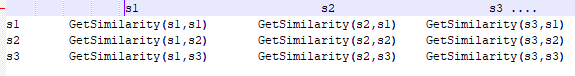# Pytorch tensor on custom calculation

I have a function GetSimilarity (sentence1, sentence2).
Now, I want to use this function in a long paragraph. Mean that I extract the paragraph into sentences, and want to measure the similarity between each sentence with the rest of the others by using my above function.
For example, the paragraph contains 10 sentences, then I will use GetSimilarity(sentence1, sentence2) and GetSimilarity (sentence1, sentence3) …and so on.I am thinking about create a 2D square matrix which columns and rows are sentences as in the illustration above.
May I ask can I use pytorch with GPU to do above calculation parallelly instead of using 2 nested loops and call the function for each pair of sentences?
Thank you so much